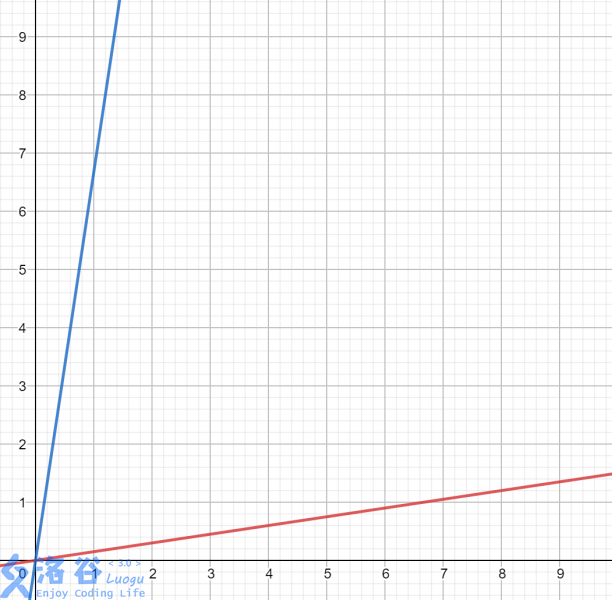# P5172 Sum 题解

## 评论

• Qingyu
等等我第一个sgn函数里面的内容写错了-。-那个1和-1的位置需要交换一下qwq
• skkyk
%
• GKxx
orz qingyu dalao tql!!!

# 做法

$$\mathrm {sgn}\; (-N)^n=\begin{cases}-1&p=2k,k\in\mathbb Z\\1&p=2k-1,k\in\mathbb Z\end{cases},\forall N>0$$

$$I=\sum^n_{d=1}1-2\left(\left\lfloor d\sqrt r\right\rfloor\bmod 2\right)$$

$$I=\sum^n_{d=1}1-2\left(\left\lfloor d\sqrt r \right\rfloor-2\left\lfloor\frac{d\sqrt r}{2}\right\rfloor\right)=n-2\sum^n_{d=1}\left\lfloor d\sqrt r\right\rfloor + 4\sum^n_{d=1}\left\lfloor\frac{\left\lfloor d\sqrt r\right\rfloor}{2}\right\rfloor$$

## 若$0 < \sqrt r = \frac{A\sqrt r+b}{c} < 1$## 评论

• 还没有评论

1. 去掉后面无理数整数部分产生的贡献。
2. 将函数关于横纵坐标翻转，然后统计新函数以下的点数，容斥求解。

$$f(a,b-\lfloor\frac{a\sqrt{r}+b}{c}\rfloor*c,c,n)$$


static int n,r;

static double R;

ll gcd(ll a,ll b){return b?gcd(b,a%b):a;}

ll getans(ll a,ll b,ll c,int n)
{
if(!n)return 0;
ll cur=gcd(a,gcd(b,c));
a/=cur,b/=cur,c/=cur;
cur=(a*R+b)/c;
ll sm=cur*n*(n+1)/2;
b-=c*cur,cur=(a*R+b)/c*n;
return sm+n*cur-getans(a*c,-b*c,a*a*r-b*b,cur);
}

inline void work()
{
}